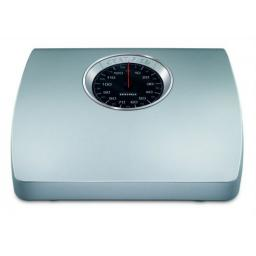# Weighs 4877

A + B weighs 75 kg, B + C weighs 83 kg, C + D weighs 92 kg. How much does A + D weigh?

x =  84 kg

### Step-by-step explanation:Did you find an error or inaccuracy? Feel free to write us. Thank you!

Tips for related online calculators
Do you have a linear equation or system of equations and looking for its solution? Or do you have a quadratic equation?